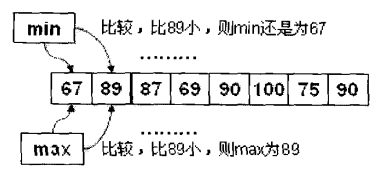# Java求数组元素的最大和最小值

```public class Example{
public static void main(String[] args) {
int score[] = { 67, 89, 87, 69, 90, 100, 75, 90 }; // 初始化数组
int max = 0; // 定义变量保存到最大值
int min = 0; // 定义变量保存到最小值
max = min = score; // 把第1个元素的内容赋值给max和min
for (int x = 0; x < score.length; x++) { // 循环求岀最大和最小
if (score[x] > max) { // 依次判断后续元素是否比max大
max = score[x]; // 如果大则修改max内容
}
if (score[x] < min) { // 依次判断后续的元素是否比min小
min = score[x]; // 如果小，则修改min内容
}
}
System.out.println("最高成绩：" + max); // 输出最大值
System.out.println("最低成绩：" + min); // 输出最小值
}
}```